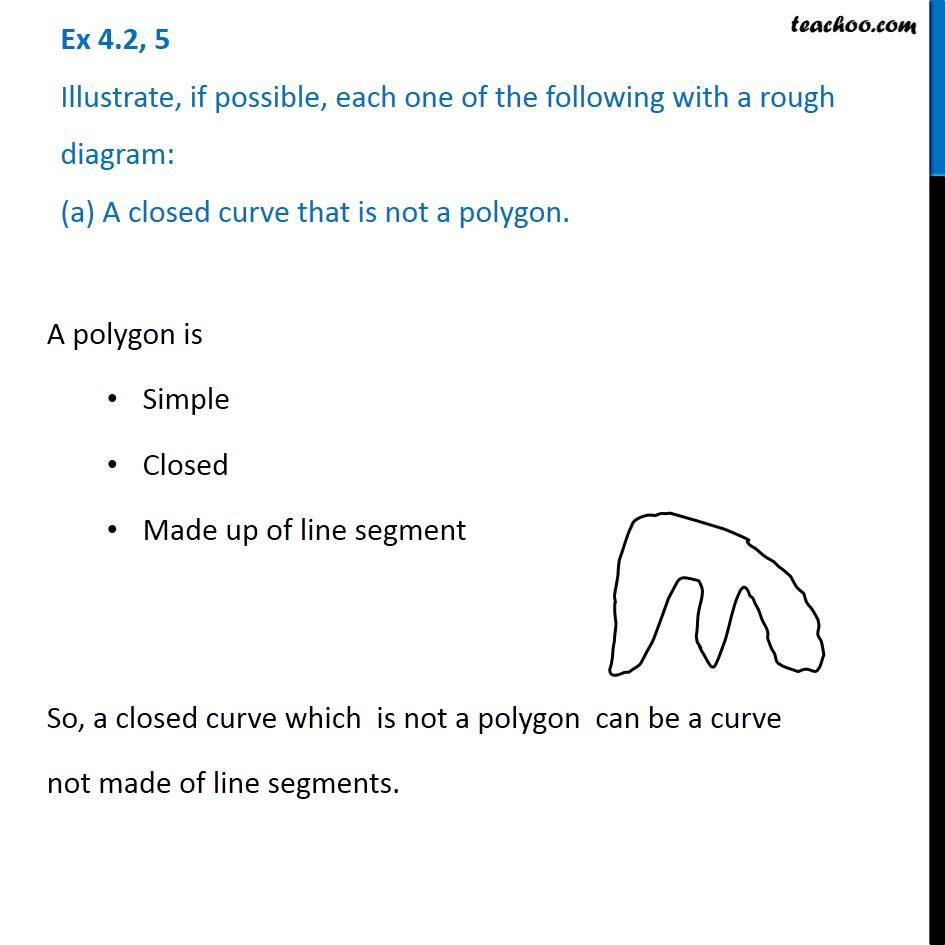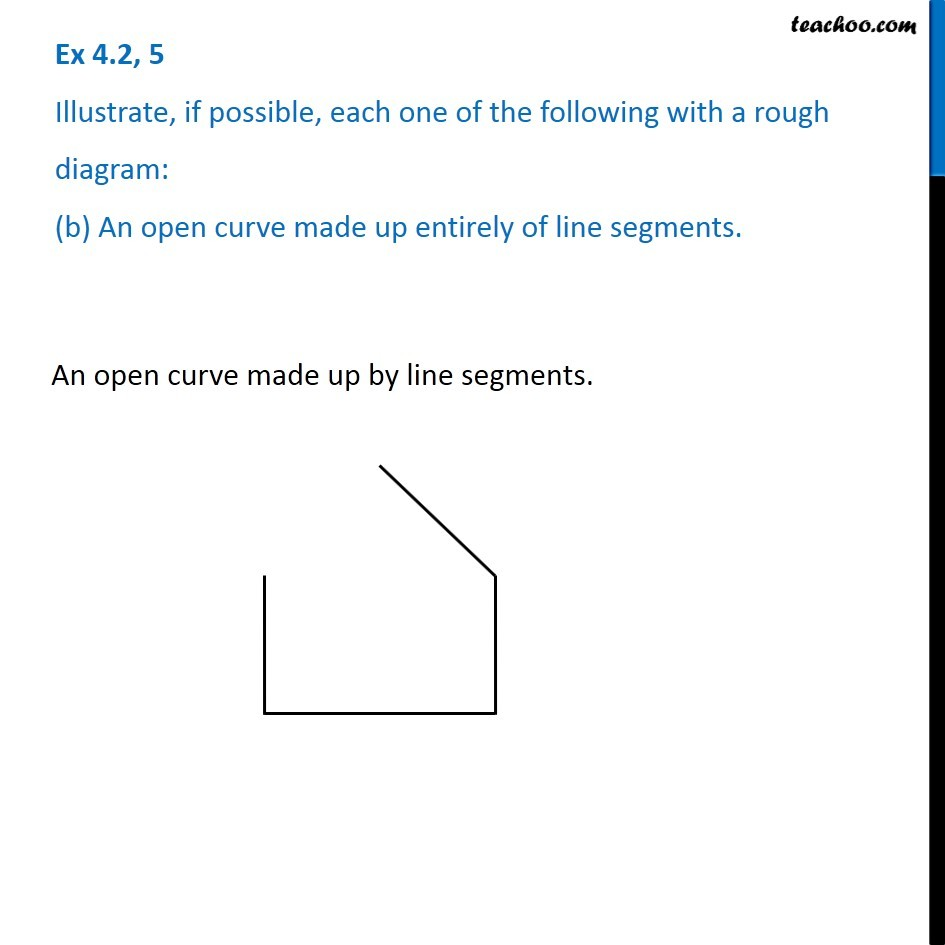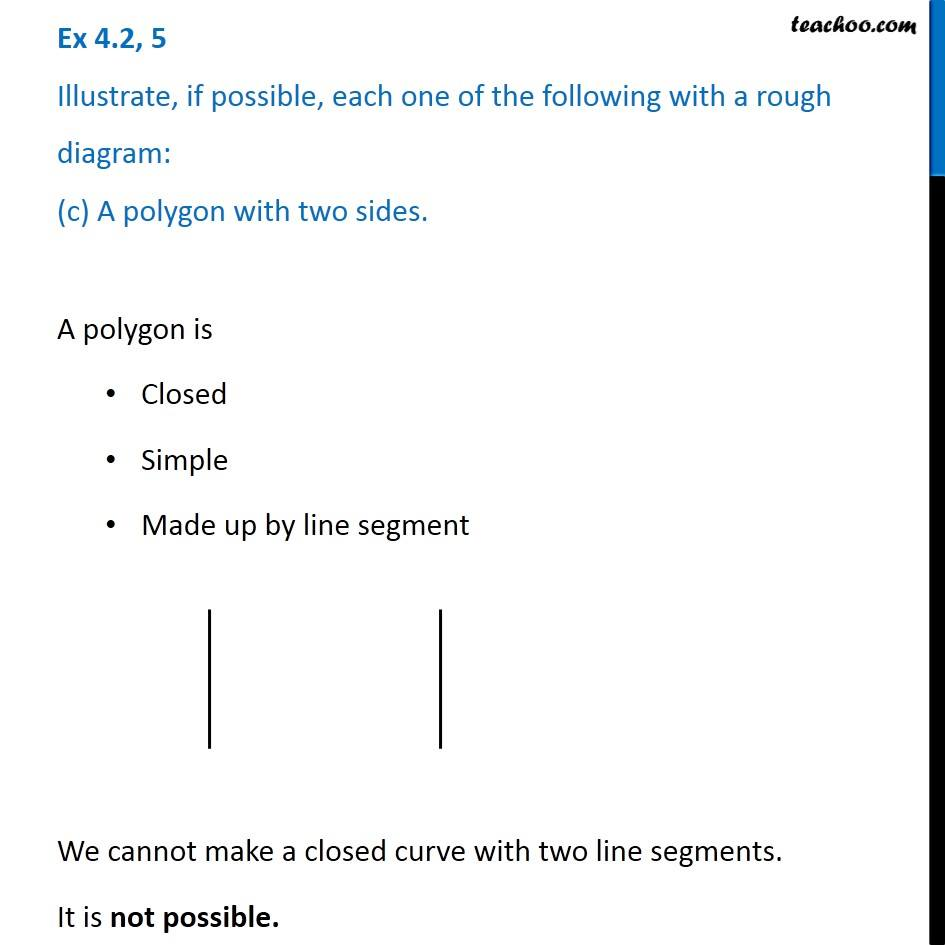Subscribe to our Youtube Channel - https://you.tube/teachoo

1. Chapter 4 Class 6 Basic Geometrical Ideas
2. Concept wise
3. Curve, Polygons

Transcript

Ex 4.2, 5 Illustrate, if possible, each one of the following with a rough diagram: (a) A closed curve that is not a polygon. A polygon is Simple Closed Made up of line segment So, a closed curve which is not a polygon can be a curve not made of line segments. Ex 4.2, 5 Illustrate, if possible, each one of the following with a rough diagram: (b) An open curve made up entirely of line segments. An open curve made up by line segments. Ex 4.2, 5 Illustrate, if possible, each one of the following with a rough diagram: (c) A polygon with two sides. A polygon is Closed Simple Made up by line segment We cannot make a closed curve with two line segments. It is not possible.

Curve, Polygons